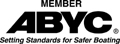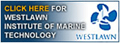Disclaimer:   I am not a spokesperson for the US Coast Guard or ABYC. For an official interpretation of regulations or standards you must contact the US Coast Guard or other organization referenced..   More.....
 I developed an E-course in Capacity and Flotation for Boat Builders, for Professional Boat Builder Magazine. They no longer offer it. I am offering it as an e-book. Go to Ike's Store to see more I also have a perfect companion to the Capacity and Flotation Course.  It is an excel spreadsheet that does the calculations to determine the amount of Flotation needed. Easy to use. Go to Ike's Store to see more

## HOW TO CALCULATE FLOTATION NEEDS

### How to calculate how much flotation your boats need.

The following are simplified methods to calculate the amount of flotation needed for a boat. The notation used, such as Boat Weight = Wb , is the same as used by ABYC, the Coast Guard and NMMA, so if you have problems you can call them and they will recognize what you're talking about. If everybody is speaking the same language it makes life a little easier.

The first method is for outboard motor boats over 2 hp. The procedure is basically the same for all boats. You need to find three things;

The boat weight and how much flotation you need to support it.

The machinery(engine) weight and how much flotation to support it.

The persons weight and how much flotation to support it.

I have simplified the calculations but in real life there is much more that goes into a boat than fiberglass and wood. Things such as screws, fittings, glue, paint, windshields, consoles, seats, etc. all add weight. There are several ways to determine the weight of each of these. The simplest way is to weight each item before you install it and add up the weights. But you need to know that different materials have different weights under water. That's what the table is for. It provides factors for determining what something weighs submerged. For instance;

Fiberglass; weighs about 1/3 under water what it weighs dry. So the factor is 0.33.

You need to determine where everything will be when the boat is swamped.

If it will be dry, use the full weight; if wet the submerged weight.

The engine will not be fully submerged on an outboard so you need to use a swamped weight. That is what it would weigh if it were only partially submerged. For that you need to take the swamped weights from the table.

Use Table 4 for outboard motor weights. You can also use Table 4-2 at the bottom of the table page for metric weights, or use ABYC Standard S-30,Outboard Engine and Related Equipment Weights Available from ABYC. If using Metric the value for water is 1000 kg/cubic meter

NOTICE:  As of June 1, 2018, Table 4 will be updated to coincide with ABYC table S-30.  In the future S-30 will be the standard for outboard engine weights. ABYC updates S-30 every five years. It will be the boat manufacturers responsibility to check for updates.

If you use S-30, the engine weights are heavier (because they are more up to date) than in the USCG table. S-30 also includes both 2 cycle and 4 cycle engines.  This results in heavier engine weights than the USCG table.  So you will come out with slightly higher amounts of flotation.

Additionally there is a different formula to calculate flotation for persons weight for boats with over 550 pounds of persons capacity.  You will see this in the examples.

Level Flotation

The following is the method for Level flotation:

Method of Calculation with example:

Factors for the Specific Gravity of materials: (Used in all calculations for Flotation)
Factor:  K
Fiberglass  =  0.33
Aluminum  = 0.63
ABS Plastic  =  0.11
Oak, white = -0.18
Oak  =  -0.56
Mahogany  =  -0.72
Plywood  = -0.81

OUTBOARD MOTORBOATS OVER 2 HP:

14 Foot outboard
40 Horsepower motor
Maximum Weight Capacity = MC = 1400 lb
Maximum Persons Capacity = PC = 900 lb
Boat Weight = 1000 lb
Use 62.4 lb per cubic foot for specific gravity of fresh water.
Use 2 lb density Foam For flotation.
Use 60.4 lb per cubic foot  for dry weight supported by 2 lb foam.

Calculate the amount of Flotation required, Ft, for The boat using:
Fb = Flotation for boat
Fp = Flotation for people
Fm = Flotation for the outboard engine

 Boat (Hull) weight(Wb) = 800 lb Hull is submerged: use submerged weights (dry weight X factor) Fiberglass = 600 lb Wood = 200 lb Fiberglass Wood Boat Weight submerged (600 X K 0.33 )+( 200 X K -0.81) Wb = 36.00 Deck is not submerged: use dry weight. Deck weight (Wd) = Fiberglas + Wood = 100lb+100 lb Wd = 200.00 Maximum Persons weight (Wp) Wp = 900.00 Swamped motor and controls + Battery Wt =  (Wm) (from col.4, 5, and 7 ABYC S-30 or 33 CFR 183.75) = 255+14+25 Wm = 314.00 Flotation for Boat Weight (Fb) = (Wb + Wd)/60.4 (cu ft of 2 lb density closed cell foam)= 36 + 200/60.4 = 236/60.4 Fb = 3.90 Dead Weight = Max Wt Capacity - Person Capacity - Motor Controls Battery wt = Motor Controls Battery Wt = From Column 6 Table 4) = 550 Dead Weight = 1400 - 900 - 459 = Wdw =(If zero or neg then Wdw = 0) Wdw = 41.00 Flotation for persons Weight (Fp) over 550 lb persons capacity = Fp F p = (0.5 X 550) + 0.125(Wp-550) + .25(Wdw) / 60.4 (1/2 the first 550 lb Persons) + (.125 remainder) + .25 dead weight 0.5 X 550)+0.125(Wp-550)+.25(Wdw)/ 60.4 = 275 + 350 + 0.25x 41 /60.4 Flotation for persons Weight (Fp Fp = 10.51 For boats with less than 550 lb persons capacity Fp = (0.25 X Wp) + .25 (Wdw) /60.4 (0.5 X Wp)+.25 (Wdw)/ 60.4= _______ + ______ / 60.4 Fp - 0.00 Flotation for swamped motor (Fm)= (Wm) / 60.4 (Wm from Table 4), 33 CFR 183 column 2) =   143 / 60.4 Fm = 2.37 TOTAL Amount of 2 lb Foam Flotation (in cubic feet) Fb + Fp + Fm = Ft =  3.9 + 10.51 + 2.37 = 16.71 Ft = 17.00 Weight of Flotation in lb.(Wfl)= 2 lb/cu ft x cu. Ft.= Ft(17.00) X 2 Wfl = 34.00 Total Boat weight = Weight of Flotation + Boat weight = Wfl + Wb

Keep track of total boat weight over a production run.  If total boat
weight increases significantly (say more than 50 lbs) you need to add
flotation.

Recalculate total Boat Weight

Weight of Flotation in pounds (Fw)=2 lb/cu ft X Cu Ft=2X 26 = Fw = 52

Total Boat weight = Weight of Flotation + Boat weight = Fw+ Wb = 52 + 800 = 852

Modified Level Flotation

The following is the method for Modified Level flotation:

OUTBOARD MOTORBOATS LESS THAN 2 HP & MANUAL PROPULSION:

See Example Calculating Capacities and Making Labels

 Fiberglass Wood Boat weight (Wb)  = ____ X K 0.33) + ( ____ X K -0.81 ) Wb = Deck weight (Wd) (if any) __________ + ___________ Wd = Maximum Persons weight (Wp) Wp = Swamped motor and controls (Wm) Wm = 27.00 (from column 2 and column 4, Table 4, 33 CFR 183.) Dead Weight (Wdw) = Max Wt Capacity - Persons Capacity Wdw = (If zero or negative ignore) Flotation for Boat Weight (Fb) = (Wb + Wd) / 60.4 (__________ + __________) /60.4 Fb =

Note: The requirement for flotation for persons weight is 2/15 of the persons weight or 0.1333 times the persons weight. But in the Guidelines the USCG uses 1/4 or 0.25 times the persons weight to provide a safety factor. This provides a safety factor of 2 or twice the amount of flotation for persons weight.  If you use 2/15 you will have a smaller amount of flotation.

Keep track of total boat weight over a production run.  If total boat
weight increases significantly (say more than 50 lbs) you need to add
flotation.

BASIC FLOTATION

The following method is for Basic Flotation:

INBOARD AND STERDRIVE MOTORBOATS:

 Fiberglass Wood Boat Weight (Wb) = ( ____ X K 0.33 ) X ( ___ x K -0.81) Wb = Deck weight (Wd) (if any) Wd = Maximum Persons Weight Wp Wp = Swamped motor, drive unit  + Battery Wt Wm = (includes motor, drive unit, propeller, shaft, battery & transmission) Dead Weight = Max Wt Capacity - total dry weight Wdw = (If zero or negative ignore) Flotation for Boat Weight (Fb) = (Wb + Wd) / 60.4 (cubic feet of 2 lb density closed cell foam) ((Wb) ___________ X (Wd) ____________ )/60.4 Fb =

Keep track of total boat weight over a production run.  If total boat
weight increases significantly (say more than 50 lb) you need to add
flotation
.